# Texas Go Math Grade 5 Lesson 5.7 Answer Key Subtraction with Renaming

Refer to our Texas Go Math Grade 5 Answer Key Pdf to score good marks in the exams. Test yourself by practicing the problems from Texas Go Math Grade 5 Lesson 5.7 Answer Key Subtraction with Renaming.

## Texas Go Math Grade 5 Lesson 5.7 Answer Key Subtraction with Renaming

Unlock the Problem

To practice for a race, Kara is running 2$$\frac{1}{2}$$ miles. When she reaches the end of her street, she knows that she has already run 1 $$\frac{5}{6}$$ miles. How many miles does Kara have left to run?

• Underline the sentence that tells you what you need to find.
• What operation should you use to solve the problem?

One Way

Subtract. 2$$\frac{1}{2}$$ – 1$$\frac{5}{6}$$

STEP 1: Estimate the difference.

STEP 2: Find a common denominator. Use the common denominator to write equivalent fractions with like denominators.

STEP 3: Rename 2$$\frac{6}{12}$$ as a mixed number with a fraction greater than 1.
Think: 2$$\frac{6}{12}$$ = 1 + 1 + $$\frac{6}{12}$$ = 1 + $$\frac{12}{12}$$ + $$\frac{6}{12}$$ = 1$$\frac{18}{12}$$
2$$\frac{6}{12}$$ = __________

STEP 4: Find the difference of the fractions. Then find the difference of the whole numbers. Write the answer in simplest form. Check to make sure your answer is reasonable.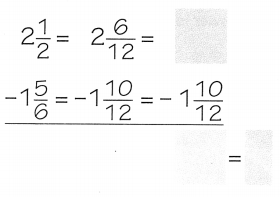So, Kara has __________ mile left to run.

STEP 1: Estimate the difference.

STEP 2: Find a common denominator. Use the common denominator to write equivalent fractions with like denominators.

STEP 3: Rename 2$$\frac{6}{12}$$ as a mixed number with a fraction greater than 1.
Think: 2$$\frac{6}{12}$$ = 1 + 1 + $$\frac{6}{12}$$ = 1 + $$\frac{12}{12}$$ + $$\frac{6}{12}$$ = 1$$\frac{18}{12}$$
2$$\frac{6}{12}$$ = 1$$\frac{18}{12}$$

STEP 4: Find the difference of the fractions. Then find the difference of the whole numbers. Write the answer in simplest form. Check to make sure your answer is reasonable.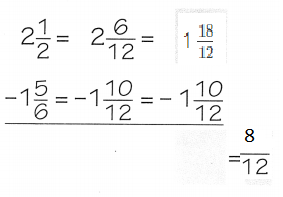So, Kara has  $$\frac{8}{12}$$ mile left to run.

Another Way
Rename both mixed numbers as fractions greater than 1.

Subtract. 2$$\frac{1}{2}$$ – 1$$\frac{5}{6}$$

STEP 1: Write equivalent fractions, using a common denominator.
A common denominator of $$\frac{1}{2}$$ and $$\frac{5}{6}$$ is 6.STEP 2: Rename both mixed numbers as fractions greater than 1.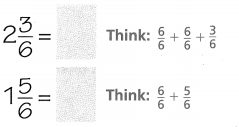STEP 3: Find the difference of the fractions. Then write the answer in simplest form.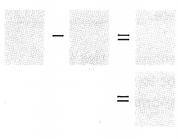2$$\frac{1}{2}$$ – 1$$\frac{5}{6}$$ = _____________

STEP 1: Write equivalent fractions, using a common denominator.
A common denominator of $$\frac{1}{2}$$ and $$\frac{5}{6}$$ is 6.STEP 2: Rename both mixed numbers as fractions greater than 1.STEP 3: Find the difference of the fractions. Then write the answer in simplest form.2$$\frac{1}{2}$$ – 1$$\frac{5}{6}$$ = $$\frac{2}{3}$$

Share and Show

Estimate. Then find the difference and write it ¡n simplest form.

Question 1.
Estimate: _____________
1$$\frac{3}{4}$$ – $$\frac{7}{8}$$
Answer: $$\frac{7}{8}$$
Explanation:
A common denominator of $$\frac{3}{4}$$ and $$\frac{7}{8}$$ is 8.
1$$\frac{3}{4}$$ = 1$$\frac{6}{8}$$ = $$\frac{8}{8}$$ +$$\frac{6}{8}$$ = $$\frac{14}{8}$$
$$\frac{7}{8}$$ =$$\frac{7}{8}$$
Find the difference of the fractions. Then write the answer in simplest form.
$$\frac{14}{8}$$ –$$\frac{7}{8}$$ = $$\frac{7}{8}$$

Question 2.
Estimate: ______________
12$$\frac{1}{9}$$ – 7$$\frac{1}{3}$$
Answer: $$\frac{43}{9}$$
Explanation:
A common denominator of $$\frac{1}{9}$$ and $$\frac{1}{3}$$ is 9.
12$$\frac{1}{9}$$ = 12$$\frac{1}{9}$$ = $$\frac{9}{9}$$  + $$\frac{9}{9}$$+ + $$\frac{9}{9}$$ + $$\frac{9}{9}$$+ $$\frac{9}{9}$$ + $$\frac{9}{9}$$ + $$\frac{9}{9}$$ + $$\frac{9}{9}$$ + $$\frac{9}{9}$$ + $$\frac{9}{9}$$+ $$\frac{9}{9}$$ + $$\frac{9}{9}$$+$$\frac{1}{9}$$ = $$\frac{109}{9}$$
7$$\frac{1}{3}$$ = $$\frac{9}{9}$$  + $$\frac{9}{9}$$+ + $$\frac{9}{9}$$ + $$\frac{9}{9}$$+ $$\frac{9}{9}$$ + $$\frac{9}{9}$$ + $$\frac{9}{9}$$ + $$\frac{3}{9}$$ =$$\frac{66}{9}$$
Find the difference of the fractions. Then write the answer in simplest form.
$$\frac{109}{9}$$–$$\frac{66}{9}$$ = $$\frac{43}{9}$$

Math Talk
Mathematical Processes

Explain the strategy you could use to solve 3$$\frac{1}{9}$$ – 2$$\frac{1}{3}$$.
Answer: $$\frac{7}{9}$$
Explanation:
A common denominator of $$\frac{1}{9}$$ and $$\frac{1}{3}$$ is 9.
3$$\frac{1}{9}$$ = $$\frac{9}{9}$$  + $$\frac{9}{9}$$+ + $$\frac{9}{9}$$ +$$\frac{1}{9}$$ = $$\frac{28}{9}$$
2$$\frac{1}{3}$$ = $$\frac{9}{9}$$  + $$\frac{9}{9}$$+ $$\frac{3}{9}$$ =$$\frac{21}{9}$$
Find the difference of the fractions. Then write the answer in simplest form.
$$\frac{28}{9}$$–$$\frac{21}{9}$$ = $$\frac{7}{9}$$

Problem Solving

Practice! Copy and Solve find the difference and write it in simplest form.

Question 3.
11$$\frac{1}{9}$$ – 3$$\frac{2}{3}$$
$$\frac{9}{9}$$  + $$\frac{9}{9}$$+ + $$\frac{9}{9}$$ + $$\frac{9}{9}$$+ $$\frac{9}{9}$$ + $$\frac{9}{9}$$ + $$\frac{9}{9}$$ + $$\frac{9}{9}$$ + $$\frac{9}{9}$$ + $$\frac{9}{9}$$+ $$\frac{9}{9}$$ + $$\frac{1}{9}$$ – $$\frac{9}{9}$$ + $$\frac{9}{9}$$+ $$\frac{9}{9}$$ + $$\frac{6}{9}$$
$$\frac{100}{9}$$ –$$\frac{33}{9}$$
$$\frac{67}{9}$$
Explanation:
STEP 1: Written the equivalent fractions, using a common denominator.
found the common denominator
STEP 2: Rename both mixed numbers as fractions greater than 1.
STEP 3: Found the difference of the fractions. Then written the answer in simplest form.

Question 4.
6 – 3$$\frac{1}{2}$$
6 – 3$$\frac{1}{2}$$
$$\frac{6}{6}$$ +$$\frac{6}{6}$$+$$\frac{6}{6}$$+$$\frac{6}{6}$$+$$\frac{6}{6}$$+$$\frac{6}{6}$$– $$\frac{6}{6}$$+$$\frac{6}{6}$$+$$\frac{6}{6}$$+$$\frac{3}{6}$$
$$\frac{36}{6}$$ – $$\frac{21}{6}$$
$$\frac{15}{6}$$
Explanation:
STEP 1: Written the equivalent fractions, using a common denominator.
found the common denominator
STEP 2: Rename both mixed numbers as fractions greater than 1.
STEP 3: Found the difference of the fractions. Then written the answer in simplest form.

Question 5.
4$$\frac{3}{8}$$ – 3$$\frac{1}{2}$$
4$$\frac{3}{8}$$ – 3$$\frac{1}{2}$$
$$\frac{8}{8}$$ +$$\frac{8}{8}$$+$$\frac{8}{8}$$+$$\frac{8}{8}$$.+$$\frac{3}{8}$$– $$\frac{8}{8}$$+$$\frac{8}{8}$$+$$\frac{8}{8}$$+$$\frac{4}{8}$$
$$\frac{35}{8}$$–$$\frac{28}{8}$$
$$\frac{7}{8}$$
Explanation:
STEP 1: Written the equivalent fractions, using a common denominator.
found the common denominator
STEP 2: Rename both mixed numbers as fractions greater than 1.
STEP 3: Found the difference of the fractions. Then written the answer in simplest form.

Question 6.
9$$\frac{1}{6}$$ – 3$$\frac{5}{8}$$
$$\frac{24}{24}$$ +$$\frac{24}{24}$$ +$$\frac{24}{24}$$ +$$\frac{24}{24}$$ +$$\frac{24}{24}$$ +$$\frac{24}{24}$$ +$$\frac{24}{24}$$ +$$\frac{24}{24}$$ +$$\frac{24}{24}$$ +$$\frac{4}{24}$$ – $$\frac{24}{24}$$ +$$\frac{24}{24}$$ +$$\frac{24}{24}$$ +$$\frac{15}{24}$$
$$\frac{220}{24}$$ – $$\frac{87}{24}$$
$$\frac{133}{24}$$
Explanation:
STEP 1: Written the equivalent fractions, using a common denominator.
found the common denominator
STEP 2: Rename both mixed numbers as fractions greater than 1.
STEP 3: Found the difference of the fractions. Then written the answer in simplest form.

Question 7.
Communicate Why is it important to write equivalent fractions before renaming? Explain.
Equivalent fractions and “fraction families” are not only used to help us add and subtract fractions with unlike denominators, but they are a big part of understanding how to simplify fractions. … This makes it very easy for students to visualize the size of each fraction and how they are related to each other.

Problem Solving

A roller coaster has 3 trains with 8 rows per train. Riders stand in rows of 4, for a total of 32 riders per train. The operators of the coaster recorded the number of riders on each train during a run. On the first train, the operators reported that 7$$\frac{1}{4}$$ rows were filled. On the second train, all 8 rows were filled, and on the third train, 5$$\frac{1}{2}$$ rows were filled.

Use the summary to solve.

Question 8.
Evaluate How many more rows were filled on the first train than on the third train?
Answer: $$\frac{7}{4}$$
Explanation:
more rows were filled on the first train than on the third train is $$\frac{7}{4}$$
7$$\frac{1}{4}$$ – 5$$\frac{1}{2}$$
$$\frac{29-12}{4}$$
$$\frac{7}{4}$$

Question 9.
H.O.T. Multi-Step How many rows were empty on the first train? How many additional riders would it take to fill the empty rows? Explain your answer.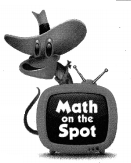Answer: $$\frac{3}{4}$$
Explanation:
$$\frac{3}{4}$$ many additional riders would it take to fill the empty rows
8-7$$\frac{1}{4}$$
8 – $$\frac{29}{4}$$

Question 10.
Multi-Step How many rows were empty on the third train? How many additional riders would it take to fill the empty rows? Explain your answer.
Answer: 2$$\frac{1}{2}$$
8 – 5$$\frac{1}{2}$$
8 – $$\frac{11}{2}$$
2$$\frac{1}{2}$$

Question 11.
You plan to enter a song writing contest. Your song must be exactly 3$$\frac{1}{2}$$ minutes long. You have a song that lasts for 4$$\frac{1}{5}$$ minutes. How many minutes do you need to cut from the song?
(A) 1$$\frac{3}{10}$$
(B) $$\frac{7}{10}$$
(C) $$\frac{3}{10}$$
(D) 1$$\frac{7}{10}$$
Explanation:
You plan to enter a song writing contest.
Your song must be exactly 3$$\frac{1}{2}$$ minutes long.
You have a song that lasts for 4$$\frac{1}{5}$$ minutes.
$$\frac{7}{10}$$ we need to cut from the song
4$$\frac{1}{5}$$ – 3$$\frac{1}{2}$$
$$\frac{27}{5}$$ – $$\frac{7}{2}$$
$$\frac{42 – 35}{10}$$
$$\frac{7}{10}$$

Question 12.
Harris and Ji are spending a weekend camping. Their campsite is 6$$\frac{1}{4}$$ kilometers from the main park road. They can take an ATV for the first 4$$\frac{7}{10}$$ kilometers, but they must walk the rest of the way. How far do Harris and Ji need to walk to get to their campsite?
(A) 1$$\frac{11}{20}$$km
(B) 1$$\frac{19}{20}$$km
(C) 2$$\frac{9}{20}$$km
(D) 2$$\frac{19}{20}$$km
Explanation:
Harris and Ji are spending a weekend camping.
Their campsite is 6$$\frac{1}{4}$$ kilometers from the main park road.
They can take an ATV for the first 4$$\frac{7}{10}$$ kilometers,
but they must walk the rest of the way.
1$$\frac{11}{20}$$km Harris and Ji need to walk to get to their campsite
$$\frac{25}{4}$$ – $$\frac{47}{10}$$
$$\frac{125}{20}$$ – $$\frac{94}{20}$$
$$\frac{31}{20}$$

Question 13.
Multi-Step Three commercials are played in a row between songs on the radio. The three commercials fill exactly 3 minutes of time. If the first commercial uses 1$$\frac{1}{6}$$ minutes, and the second uses $$\frac{3}{5}$$ minute, how long is the third commercial?
(A) $$\frac{23}{30}$$ minute
(B) 1$$\frac{23}{30}$$ minutes
(C) 1$$\frac{7}{30}$$ minutes
(D) 2$$\frac{7}{30}$$ minutes
Explanation:
Three commercials are played in a row between songs on the radio.
The three commercials fill exactly 3 minutes of time.
If the first commercial uses 1$$\frac{1}{6}$$ minutes,
and the second uses $$\frac{3}{5}$$ minute,
1$$\frac{1}{6}$$ + $$\frac{3}{5}$$  – 3
$$\frac{30}{30}$$+$$\frac{5}{30}$$+$$\frac{18}{30}$$ –$$\frac{30}{30}$$+$$\frac{30}{30}$$+$$\frac{30}{30}$$
$$\frac{23}{30}$$ – $$\frac{60}{30}$$
$$\frac{37}{30}$$

Texas Test Prep

Question 14.
Coach Lopes filled a water cooler with 4$$\frac{1}{2}$$ gallons of water before a game. At the end of the game, 1$$\frac{3}{4}$$ gallons of water were left over. How many gallons of water did the team drink during the game?
(A) 3$$\frac{1}{4}$$ gallons
(B) 2$$\frac{1}{2}$$ gallons
(C) 2$$\frac{3}{4}$$ gallons
(D) $$\frac{3}{4}$$ gallon
Explanation:
Coach Lopes filled a water cooler with 4$$\frac{1}{2}$$ gallons of water before a game.
At the end of the game, 1$$\frac{3}{4}$$ gallons of water were left over.
2$$\frac{3}{4}$$ gallons of water the team drink during the game.
4$$\frac{1}{2}$$ – 2$$\frac{3}{4}$$
$$\frac{9}{2}$$– $$\frac{7}{4}$$
$$\frac{18}{7}$$
$$\frac{11}{4}$$

### Texas Go Math Grade 5 Lesson 5.7 Homework and Practice Answer Key

Find the difference and write it in simplest form.

Question 1.
5$$\frac{1}{2}$$ – 1$$\frac{2}{3}$$ ____________
5$$\frac{1}{2}$$ – 1$$\frac{2}{3}$$
$$\frac{6}{6}$$ +$$\frac{6}{6}$$+$$\frac{6}{6}$$+$$\frac{6}{6}$$+$$\frac{6}{6}$$+$$\frac{2}{6}$$–$$\frac{6}{6}$$+$$\frac{1}{6}$$
$$\frac{25}{6}$$ – $$\frac{2}{6}$$
$$\frac{23}{6}$$
Explanation:
STEP 1: Written the equivalent fractions, using a common denominator.
found the common denominator
STEP 2: Rename both mixed numbers as fractions greater than 1.
STEP 3: Found the difference of the fractions. Then written the answer in simplest form.

Question 2.
4$$\frac{2}{9}$$ – 3$$\frac{1}{3}$$ ____________
4$$\frac{2}{9}$$ – 3$$\frac{1}{3}$$
$$\frac{9}{9}$$ +$$\frac{9}{9}$$+$$\frac{9}{9}$$+$$\frac{9}{9}$$+$$\frac{2}{9}$$ – $$\frac{9}{9}$$+$$\frac{9}{9}$$+$$\frac{9}{9}$$+$$\frac{3}{9}$$
$$\frac{11}{9}$$ – $$\frac{3}{9}$$
$$\frac{8}{9}$$
Explanation:
STEP 1: Written the equivalent fractions, using a common denominator.
found the common denominator
STEP 2: Rename both mixed numbers as fractions greater than 1.
STEP 3: Found the difference of the fractions. Then written the answer in simplest form.

Question 3.
8 – 3$$\frac{2}{7}$$ _____________
$$\frac{7}{7}$$+$$\frac{7}{7}$$+$$\frac{7}{7}$$+$$\frac{7}{7}$$$$\frac{7}{7}$$+$$\frac{7}{7}$$+$$\frac{7}{7}$$–$$\frac{7}{7}$$+$$\frac{7}{7}$$$$\frac{7}{7}$$+$$\frac{2}{7}$$
$$\frac{35}{7}$$–$$\frac{2}{7}$$
$$\frac{33}{7}$$
Explanation:
STEP 1: Written the equivalent fractions, using a common denominator.
found the common denominator
STEP 2: Rename both mixed numbers as fractions greater than 1.
STEP 3: Found the difference of the fractions. Then written the answer in simplest form.

Question 4.
7$$\frac{2}{5}$$ – 2$$\frac{1}{2}$$ _____________
7$$\frac{2}{5}$$ – 2$$\frac{1}{2}$$
$$\frac{10}{10}$$ +$$\frac{10}{10}$$+$$\frac{10}{10}$$+$$\frac{10}{10}$$+$$\frac{10}{10}$$+$$\frac{10}{10}$$+$$\frac{10}{10}$$+$$\frac{4}{10}$$–$$\frac{10}{10}$$+$$\frac{10}{10}$$+$$\frac{5}{10}$$
$$\frac{54}{10}$$–$$\frac{5}{10}$$
$$\frac{49}{10}$$
Explanation:
STEP 1: Written the equivalent fractions, using a common denominator.
found the common denominator
STEP 2: Rename both mixed numbers as fractions greater than 1.
STEP 3: Found the difference of the fractions. Then written the answer in simplest form.

Question 5.
4$$\frac{2}{3}$$ – 2$$\frac{5}{6}$$ _____________
4$$\frac{2}{3}$$ – 2$$\frac{5}{6}$$
$$\frac{6}{6}$$+$$\frac{6}{6}$$+$$\frac{6}{6}$$+$$\frac{6}{6}$$+$$\frac{4}{6}$$–$$\frac{6}{6}$$+$$\frac{6}{6}$$+$$\frac{5}{6}$$
$$\frac{16}{6}$$–$$\frac{5}{6}$$
$$\frac{11}{6}$$
Explanation:
STEP 1: Written the equivalent fractions, using a common denominator.
found the common denominator
STEP 2: Rename both mixed numbers as fractions greater than 1.
STEP 3: Found the difference of the fractions. Then written the answer in simplest form.

Question 6.
8$$\frac{3}{10}$$ – 5$$\frac{3}{5}$$ ____________
8$$\frac{3}{10}$$ – 5$$\frac{3}{5}$$
$$\frac{10}{10}$$ +$$\frac{10}{10}$$+$$\frac{10}{10}$$+$$\frac{10}{10}$$+$$\frac{10}{10}$$+$$\frac{10}{10}$$+$$\frac{10}{10}$$+$$\frac{10}{10}$$+$$\frac{3}{10}$$–$$\frac{10}{10}$$+$$\frac{10}{10}$$+$$\frac{10}{10}$$+$$\frac{10}{10}$$+$$\frac{6}{10}$$
$$\frac{33}{10}$$–$$\frac{6}{10}$$
$$\frac{27}{10}$$
Explanation:
STEP 1: Written the equivalent fractions, using a common denominator.
found the common denominator
STEP 2: Rename both mixed numbers as fractions greater than 1.
STEP 3: Found the difference of the fractions. Then written the answer in simplest form.

Question 7.
4$$\frac{1}{8}$$ – 1$$\frac{1}{2}$$ ____________
4$$\frac{1}{8}$$ – 1$$\frac{1}{2}$$
$$\frac{8}{8}$$+$$\frac{8}{8}$$+$$\frac{8}{8}$$+$$\frac{8}{8}$$+$$\frac{1}{8}$$–$$\frac{8}{8}$$+$$\frac{4}{8}$$
$$\frac{25}{8}$$–$$\frac{4}{8}$$
$$\frac{21}{8}$$
Explanation:
STEP 1: Written the equivalent fractions, using a common denominator.
found the common denominator
STEP 2: Rename both mixed numbers as fractions greater than 1.
STEP 3: Found the difference of the fractions. Then written the answer in simplest form.

Question 8.
6$$\frac{5}{12}$$ – 5$$\frac{3}{4}$$ ___________
6$$\frac{5}{12}$$ – 5$$\frac{3}{4}$$
$$\frac{12}{12}$$ +$$\frac{5}{12}$$ –$$\frac{9}{12}$$
$$\frac{17}{12}$$–$$\frac{9}{12}$$
$$\frac{8}{12}$$
Explanation:
STEP 1: Written the equivalent fractions, using a common denominator.
found the common denominator
STEP 2: Rename both mixed numbers as fractions greater than 1.
STEP 3: Found the difference of the fractions. Then written the answer in simplest form.

Question 9.
12$$\frac{1}{6}$$ – 4$$\frac{3}{8}$$ ____________
8 x 24 + $$\frac{4}{24}$$ –$$\frac{9}{24}$$
$$\frac{196}{24}$$ –$$\frac{9}{24}$$
$$\frac{187}{24}$$
Explanation:
STEP 1: Written the equivalent fractions, using a common denominator.
found the common denominator
STEP 2: Rename both mixed numbers as fractions greater than 1.
STEP 3: Found the difference of the fractions. Then written the answer in simplest form.

Question 10.
9$$\frac{1}{6}$$ – 3$$\frac{4}{5}$$ ___________
9$$\frac{1}{6}$$ – 3$$\frac{4}{5}$$
6 x 30 + $$\frac{5}{30}$$ –$$\frac{24}{30}$$
$$\frac{185}{30}$$ –$$\frac{24}{30}$$
$$\frac{161}{30}$$
Explanation:
STEP 1: Written the equivalent fractions, using a common denominator.
found the common denominator
STEP 2: Rename both mixed numbers as fractions greater than 1.
STEP 3: Found the difference of the fractions. Then written the answer in simplest form.

Question 11.
13$$\frac{3}{5}$$ – 4$$\frac{3}{4}$$ __________
13$$\frac{3}{5}$$ – 4$$\frac{3}{4}$$
9 x 20 + $$\frac{12}{20}$$ – $$\frac{15}{20}$$
$$\frac{192}{20}$$ – $$\frac{15}{20}$$
$$\frac{177}{20}$$
Explanation:
STEP 1: Written the equivalent fractions, using a common denominator.
found the common denominator
STEP 2: Rename both mixed numbers as fractions greater than 1.
STEP 3: Found the difference of the fractions. Then written the answer in simplest form.

Question 12.
6$$\frac{3}{8}$$ – 2$$\frac{5}{9}$$ __________
6$$\frac{3}{8}$$ – 2$$\frac{5}{9}$$
4 x 72 + $$\frac{27}{72}$$ – $$\frac{40}{72}$$
$$\frac{315}{72}$$– $$\frac{40}{72}$$
$$\frac{275}{72}$$
Explanation:
STEP 1: Written the equivalent fractions, using a common denominator.
found the common denominator
STEP 2: Rename both mixed numbers as fractions greater than 1.
STEP 3: Found the difference of the fractions. Then written the answer in simplest form.

Question 13.
2$$\frac{1}{3}$$ – 1$$\frac{5}{6}$$ ___________
2$$\frac{1}{3}$$ – 1$$\frac{5}{6}$$
1 x6 +$$\frac{2}{6}$$ – $$\frac{5}{6}$$
$$\frac{8}{6}$$–$$\frac{5}{6}$$
$$\frac{3}{6}$$
Explanation:
STEP 1: Written the equivalent fractions, using a common denominator.
found the common denominator
STEP 2: Rename both mixed numbers as fractions greater than 1.
STEP 3: Found the difference of the fractions. Then written the answer in simplest form.

Question 14.
5 – 2$$\frac{1}{2}$$ ___________
5 – 2$$\frac{1}{2}$$
$$\frac{2}{2}$$+$$\frac{2}{2}$$+$$\frac{2}{2}$$+$$\frac{2}{2}$$+$$\frac{2}{2}$$–$$\frac{2}{2}$$+$$\frac{2}{2}$$+$$\frac{1}{2}$$
$$\frac{6}{2}$$–$$\frac{1}{2}$$
$$\frac{5}{2}$$
Explanation:
STEP 1: Written the equivalent fractions, using a common denominator.
found the common denominator
STEP 2: Rename both mixed numbers as fractions greater than 1.
STEP 3: Found the difference of the fractions. Then written the answer in simplest form.

Question 15.
1$$\frac{1}{10}$$ – $$\frac{1}{2}$$ ___________
1$$\frac{1}{10}$$ – $$\frac{1}{2}$$
$$\frac{10}{10}$$+$$\frac{1}{10}$$ – $$\frac{5}{10}$$
$$\frac{6}{10}$$
Explanation:
STEP 1: Written the equivalent fractions, using a common denominator.
found the common denominator
STEP 2: Rename both mixed numbers as fractions greater than 1.
STEP 3: Found the difference of the fractions. Then written the answer in simplest form.

Question 16.
7$$\frac{1}{4}$$ – 1$$\frac{3}{8}$$ ___________
7$$\frac{1}{4}$$ – 1$$\frac{3}{8}$$
6 x 8 +$$\frac{2}{8}$$–$$\frac{3}{8}$$
$$\frac{50}{8}$$–$$\frac{3}{8}$$
$$\frac{47}{8}$$
Explanation:
STEP 1: Written the equivalent fractions, using a common denominator.
found the common denominator
STEP 2: Rename both mixed numbers as fractions greater than 1.
STEP 3: Found the difference of the fractions. Then written the answer in simplest form.

Question 17.
Tell how you know when you need to use renaming when subtracting mixed numbers.

Problem Solving

Use the table for 20-21.

Question 18.
Chef Rossi makes 7$$\frac{5}{8}$$ gallons of soup for the soup kitchen. She needs to fill a large container with a capacity of 9$$\frac{1}{2}$$ gallons. How many more gallons of soup does Chef Rassi need to make?
Answer: $$\frac{15}{8}$$
Explanation:
Chef Rossi makes 7$$\frac{5}{8}$$ gallons of soup for the soup kitchen.
She needs to fill a large container with a capacity of 9$$\frac{1}{2}$$ gallons.
Chef Rassi need to make 1$$\frac{7}{8}$$  more gallons of soup
9$$\frac{1}{2}$$ – 7$$\frac{5}{8}$$
$$\frac{61-76}{8}$$
$$\frac{15}{8}$$

Question 19.
Derek made a rope swing with a length of 5$$\frac{3}{4}$$ feet. Nick’s rope swing is 6$$\frac{1}{8}$$ feet long. How much longer is Nick’s swing than Derek’s swing?
Answer: $$\frac{3}{8}$$
Explanation:
Derek made a rope swing with a length of 5$$\frac{3}{4}$$ feet.
Nick’s rope swing is 6$$\frac{1}{8}$$ feet long.
$$\frac{3}{8}$$ longer is Nick’s swing than Derek’s swing
6$$\frac{1}{8}$$ – 5$$\frac{3}{4}$$
$$\frac{49}{8}$$ – $$\frac{46}{8}$$
$$\frac{3}{8}$$

Lesson Check

Use the table for 20 – 21.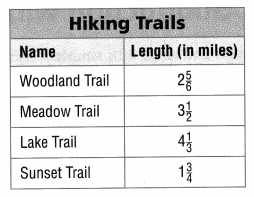Question 20.
Sasha and Lee are looking at the park’s list of hiking trails in order to choose a hike. How much farther will they have to hike if they choose Lake Trail instead of Woodland Trail?
(A) 4$$\frac{1}{3}$$ miles
(B) 2$$\frac{2}{3}$$ miles
(C) 2$$\frac{1}{2}$$ miles
(D) 1$$\frac{1}{2}$$ miles
Explanation:
Sasha and Lee are looking at the park’s list of hiking trails in order to choose a hike.
4$$\frac{1}{3}$$ miles farther  they have to hike if they choose Lake Trail instead of Woodland Trail
4$$\frac{1}{3}$$ – 2$$\frac{5}{6}$$
$$\frac{26-17}{6}$$

Question 21.
Lee and Sasha have hiked $$\frac{7}{8}$$ mile on Meadow Trail. How much farther do they need to hike to get to the end of the trail?
(A) 2$$\frac{5}{8}$$ miles
(B) 4 miles
(C) 4$$\frac{3}{8}$$ miles
(D) $$\frac{3}{8}$$ mile
Explanation:
Lee and Sasha have hiked $$\frac{7}{8}$$ mile on Meadow Trail.
2$$\frac{5}{8}$$ miles fartherthey need to hike to get to the end of the trail
3$$\frac{1}{2}$$ – $$\frac{7}{8}$$
$$\frac{28-7}{8}$$
$$\frac{21}{8}$$

Question 22.
Mario renames the mixed numbers to fractions greater than 1 to find 4$$\frac{1}{2}$$ – 2$$\frac{2}{3}$$. Which fractions should Mario use to find the difference?
(A) $$\frac{27}{6}$$, $$\frac{16}{6}$$
(B) $$\frac{24}{6}$$, $$\frac{12}{6}$$
(C) $$\frac{27}{5}$$, $$\frac{16}{5}$$
(D) $$\frac{7}{6}$$, $$\frac{8}{6}$$
Mario renames the mixed numbers to fractions greater than 1 to find
4$$\frac{1}{2}$$ – 2$$\frac{2}{3}$$.
$$\frac{27}{6}$$, $$\frac{16}{6}$$ Mario used to find the difference
4$$\frac{1}{2}$$ – 2$$\frac{2}{3}$$.
$$\frac{9}{2}$$,$$\frac{8}{3}$$.
is renamed as
$$\frac{27}{6}$$, $$\frac{16}{6}$$

Question 23.
Multi-Step Ian’s mother drives 8$$\frac{1}{5}$$ miles to work each day. His father drives 9$$\frac{1}{2}$$ miles round-trip between home and work. How much farther is Ian’s mother’s round-trip than his father’s?
(A) 6$$\frac{9}{10}$$ miles
(B) 16$$\frac{2}{5}$$ miles
(C) 7$$\frac{1}{10}$$ miles
(D) 17$$\frac{7}{10}$$ miles
8$$\frac{1}{5}$$ x 2
= 16$$\frac{2}{5}$$ miles
$$\frac{82}{5}$$ – $$\frac{19}{2}$$
$$\frac{164-95}{10}$$
= 6$$\frac{9}{10}$$ miles
Ian’s mother drives 8$$\frac{1}{5}$$ miles to work each day.
His father drives 9$$\frac{1}{2}$$ miles round-trip between home and work.
6$$\frac{9}{10}$$ miles farther is Ian’s mother’s round-trip than his father’s.

Question 24.
Multi-Step Mrs. Holbrook’s delivery truck consumes 12 galLons of gasoline in three days. If 2$$\frac{4}{5}$$ gallons of gas are consumed on the first day, and 3$$\frac{7}{10}$$ gallons are consumed on the second day, how much is consumed on the third day?
(A) 6$$\frac{1}{2}$$ gallons
(B) 9$$\frac{1}{5}$$ gallons
(C) 5$$\frac{1}{2}$$ gallons
(D) 8$$\frac{3}{10}$$ gallons
If 2$$\frac{4}{5}$$ gallons of gas are consumed on the first day,
and 3$$\frac{7}{10}$$ gallons are consumed on the second day,
5$$\frac{1}{2}$$ gallons of gas on third day
12 -2$$\frac{4}{5}$$  + 3$$\frac{7}{10}$$
12 – $$\frac{28+37}{10}$$
12 – $$\frac{65}{10}$$
5$$\frac{1}{2}$$ gallons